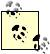# Computing Present Value

Problem

You need to determine the present value of a future dollar amount, considering the time value of money.

Solution

Use the built-in function PV.

Discussion

Consider this example: what is the present value of a \$5,000 payment to occur three years from now if the annual interest rate is 7%? You can use the present value, PV, function to find out. The syntax for PV is =PV(rate, nper, pmt, fv, type), where rate is the interest rate per period, nper is the number of payment periods, pmt is the payment per period, fv is the future value, and type is an indicator of the payment type (0 for payments at the end of each pay period or 1 for payments at the beginning of each pay period).You need to keep the interest rate and pay periods consistent when using this formula. For example, if you're using a 7% annual interest rate, then your pay period is in years. If your payments are each month, then you need to use the monthly interest rate.

To compute the present value for our example, enter the formula =PV(0.07, 3, 0, 5000) in a cell. The result comes to -\$4,081.49. Excel generates this as a negative number, implying that this is what you would have to invest now at the given rate to have a future value of \$5,000 three years from now. You can get rid of the negative by taking the absolute value, using the ABS function (see Chapter 7).

Take a look at the other recipes in this chapter to learn about other calculations of the time value of money.Excel Scientific and Engineering Cookbook (Cookbooks (OReilly))
ISBN: 0596008791
EAN: 2147483647
Year: N/A
Pages: 206
Authors: David M Bourg